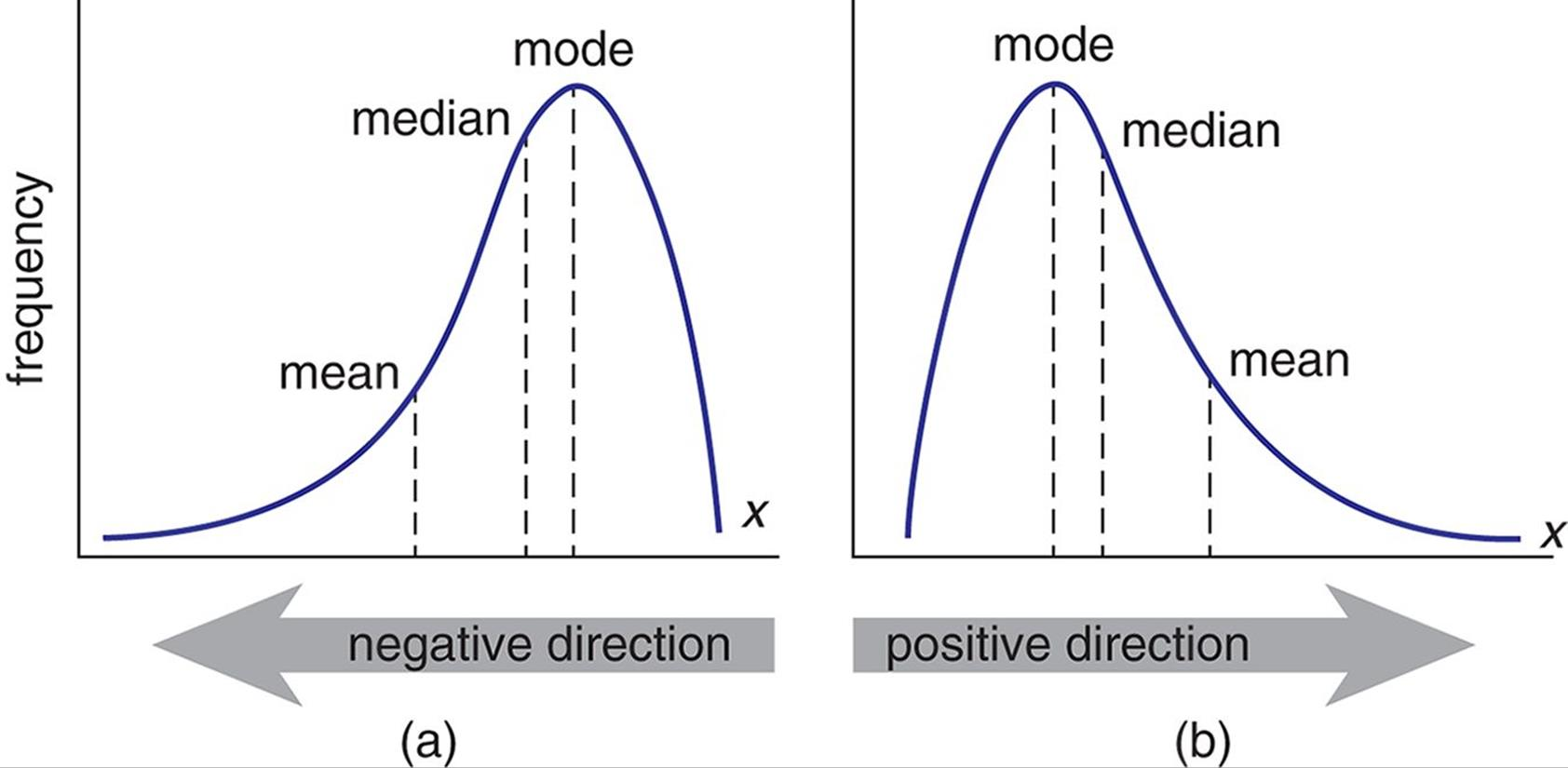/  Top Machine learning interview questions and answers   /  What is the importance of Mean, Variance, Standard Deviation in identifying details of particular feature in Dataset? How do you calculate it?## What is the importance of Mean, Variance, Standard Deviation in identifying details of particular feature in Dataset? How do you calculate it?

Mean is average of a given set of data.Variance is the sum of squares of differences between all numbers and means.Standard Deviation is square root of variance. It is a measure of the extent to which data varies from the mean.